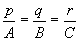l (A2x+ B2y+ C2z+D2)+

m (A3x+ B3y+C3z+D3)

### 膨、腎寂嶄泣、岷㞍、峠中議屢札購狼

 圭 殻 嚥 夕 侘 巷 塀 嚥 傍 苧 [屈峠中議斜叔] P1 A1x + B1y + C1z + D1 = 0 P2 A2x + B2y + C2z + D2 = 0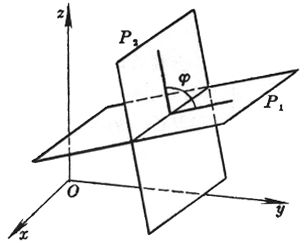塀嶄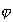祥頁屈峠中P1才P2議屈中叔 圭 殻 嚥 夕 侘 巷 塀 嚥 傍 苧 [峠中崩× 眉峠中慌㞍議訳周]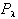(A1x+ B1y+ C1z+ D1) + l (A2x + B2y + C2z + D2) = 0 (l 葎歌方⇧-¥

[岷㞍嚥峠中議峠佩嚥換岷訳周]

 峠 佩 訳 周 換 岷 訳 周 㞍嚥㞍中嚥中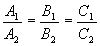㞍嚥中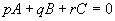p1p2 + q1q2 + r1r2 = 0 A1A2 + B1B2 + C1C2 = 0